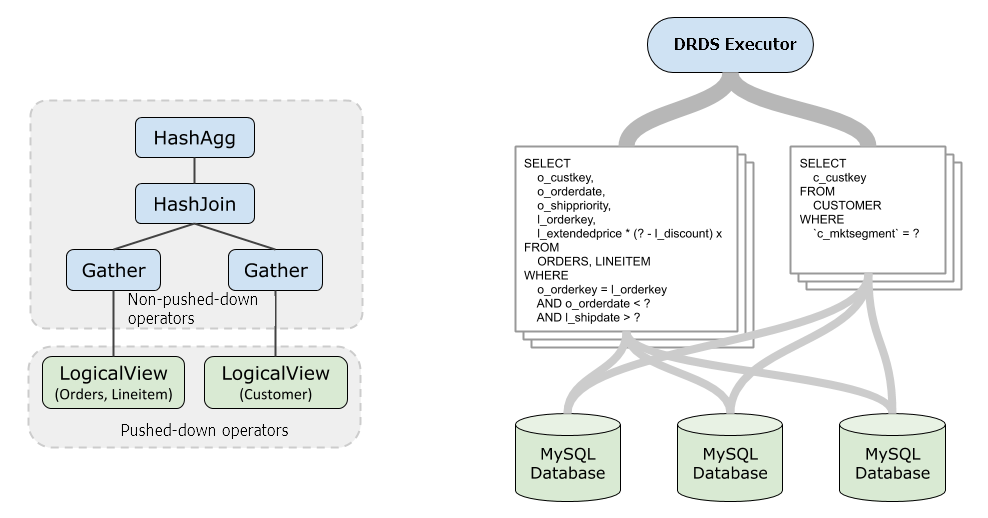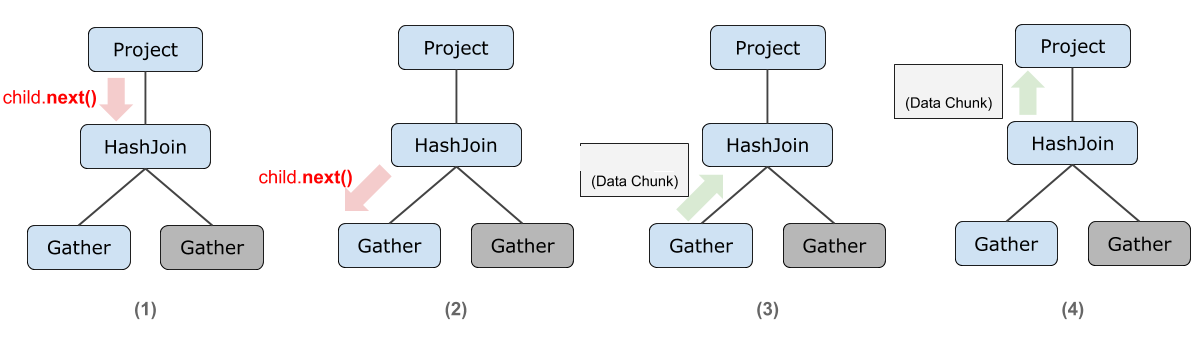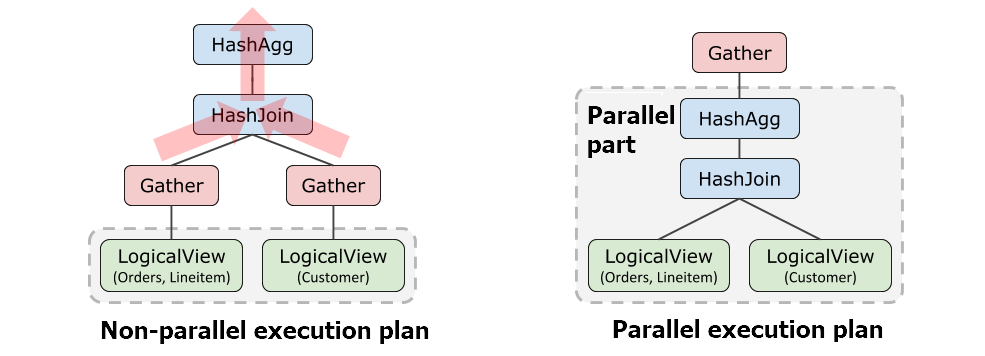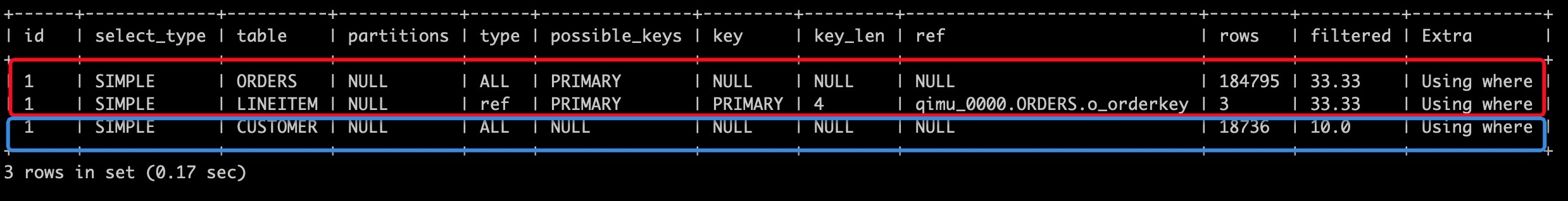This topic describes how the SQL executor of DRDS executes the Structured Query Language (SQL) parts that cannot be pushed down.

## Basic concepts

The SQL executor is a component that executes the operators at the logical layer in DRDS. Simple SQL statements for point queries can often be entirely pushed down to the storage layer MySQL for execution. Therefore, you are not aware of the existence of the executor. The results of MySQL are transmitted back to the user after they are decoded and encoded. However, operators in complex SQL statements cannot all be pushed down. In this case, the DRDS executor is required to execute the computations that cannot be pushed down.

The following query SQL statement is used as an example:

``````SELECT l_orderkey, sum(l_extendedprice *(1 - l_discount)) AS revenue
FROM CUSTOMER, ORDERS, LINEITEM
WHERE c_mktsegment = 'AUTOMOBILE'
and c_custkey = o_custkey
and l_orderkey = o_orderkey
and o_orderdate < '1995-03-13'
and l_shipdate > '1995-03-13'
GROUP BY l_orderkey;``````

Run the `EXPLAIN` command to view the execution plan of DRDS.

``````HashAgg(group="l_orderkey", revenue="SUM(*)")
HashJoin(condition="o_custkey = c_custkey", type="inner")
Gather(concurrent=true)
LogicalView(tables="ORDERS_[0-7],LINEITEM_[0-7]", shardCount=8, sql="SELECT `ORDERS`.`o_custkey`, `LINEITEM`.`l_orderkey`, (`LINEITEM`.`l_extendedprice` * (? - `LINEITEM`.`l_discount`)) AS `x` FROM `ORDERS` AS `ORDERS` INNER JOIN `LINEITEM` AS `LINEITEM` ON (((`ORDERS`.`o_orderkey` = `LINEITEM`.`l_orderkey`) AND (`ORDERS`.`o_orderdate` < ?)) AND (`LINEITEM`.`l_shipdate` > ?))")
Gather(concurrent=true)
LogicalView(tables="CUSTOMER_[0-7]", shardCount=8, sql="SELECT `c_custkey` FROM `CUSTOMER` AS `CUSTOMER` WHERE (`c_mktsegment` = ?)")``````

In the following figure, the SQL statement of `LogicalView` is delivered to MySQL when the SQL statement is executed. The parts (operators other than `LogicalView`) that cannot be pushed down are computed by the DRDS executor. This way, the required final results of the SQL statement of the user are retrieved.## Volcano model

Like a number of databases, DRDS adopts the Volcano model. API operations, such as `open()` and `next()`, are defined for all the operators. Operators form an operator tree based on the execution plan. An upper-layer operator is computed by calling the output result of the `next()` operation of a lower-layer operator. In the end, the top-layer operator generates the desired results for the user and returns the results to the client.

In the following example, assume that the `HashJoin` operator has created a hash table. When the upper-layer Project operator requests data, `HashJoin` first requests a batch of data from the lower-layer `Gather` operator. Then, HashJoin looks up the table to retrieve the JOIN result and returns the result to the Project operator.In some cases, an operator needs to read all the data and cache the data in the memory. This process is called materialization. For example, the HashJoin operator needs to read all the data from the inner table and create a hash table in the memory. Other similar operators include HashAgg (aggregation) and MemSort (sorting).

Memory resources are limited. Therefore, if the amount of materialized data exceeds the limit on a single query or the total used memory exceeds the memory limit of the DRDS node, an out of memory error (`OUT_OF_MEMORY`) is reported.

## Parallel queries

A parallel query refers to a complex query that is executed in parallel by using multiple threads.

Note This feature is available in only DRDS Standard Edition and Enterprise Edition. The feature is unavailable in Starter Edition due to the limits of hardware specifications.

The execution plans of parallel queries are changed compared with the original execution plans. The preceding query is also used as an example. The following example shows the parallel execution plan of the preceding query:

``````Gather(parallel=true)
ParallelHashAgg(group="o_orderdate,o_shippriority,l_orderkey", revenue="SUM(*)")
ParallelHashJoin(condition="o_custkey = c_custkey", type="inner")
LogicalView(tables="ORDERS_[0-7],LINEITEM_[0-7]", shardCount=8, sql="SELECT `ORDERS`.`o_custkey`, `ORDERS`.`o_orderdate`, `ORDERS`.`o_shippriority`, `LINEITEM`.`l_orderkey`, (`LINEITEM`.`l_extendedprice` * (? - `LINEITEM`.`l_discount`)) AS `x` FROM `ORDERS` AS `ORDERS` INNER JOIN `LINEITEM` AS `LINEITEM` ON (((`ORDERS`.`o_orderkey` = `LINEITEM`.`l_orderkey`) AND (`ORDERS`.`o_orderdate` < ?)) AND (`LINEITEM`.`l_shipdate` > ?))", parallel=true)
LogicalView(tables="CUSTOMER_[0-7]", shardCount=8, sql="SELECT `c_custkey` FROM `CUSTOMER` AS `CUSTOMER` WHERE (`c_mktsegment` = ?)", parallel=true)``````You can see that the Gather operator is pulled up to a higher layer in the parallel execution plan. This indicates that all the operators below the Gather operator are executed in parallel and can be aggregated until the Gather operator is reached.

The operators below Gather are instantiated to multiple execution instances during execution. Each instance corresponds to a degree of parallelism (DOP). By default, the DOP is equal to the number of cores on a single server. The default DOP of Standard Edition instances is 8. The default DOP of Enterprise Edition instances is 16.

## Diagnostic analysis of the execution process

In addition to the EXPLAIN instruction that is described in the preceding section, the following instructions can help you analyze performance issues:

• The `EXPLAIN ANALYZE` instruction analyzes the execution performance metrics of each operator in DRDS servers.
• The `EXPLAIN EXECUTE` instruction returns the EXPLAIN results of MySQL after the results are aggregated.

The following section uses the query that is provided in the preceding section as an example to describe how to analyze the performance issues of a query.

The following result (some irrelevant information has been deleted) is obtained after `EXPLAIN ANALYZE` is executed:

``````explain analyze select l_orderkey, sum(l_extendedprice *(1 - l_discount)) as revenue from CUSTOMER, ORDERS, LINEITEM where c_mktsegment = 'AUTOMOBILE' and c_custkey = o_custkey and l_orderkey = o_orderkey and o_orderdate < '1995-03-13' and l_shipdate > '1995-03-13' group by l_orderkey;

HashAgg(group="o_orderdate,o_shippriority,l_orderkey", revenue="SUM(*)")
... actual time = 23.916 + 0.000, actual rowcount = 11479, actual memory = 1048576, instances = 1 ...
HashJoin(condition="o_custkey = c_custkey", type="inner")
... actual time = 0.290 + 23.584, actual rowcount = 30266, actual memory = 1048576, instances = 1 ...
Gather(concurrent=true)
... actual time = 0.000 + 23.556, actual rowcount = 151186, actual memory = 0, instances = 1 ...
LogicalView(tables="ORDERS_[0-7],LINEITEM_[0-7]", shardCount=8, sql="SELECT `ORDERS`.`o_custkey`, `ORDERS`.`o_orderdate`, `ORDERS`.`o_shippriority`, `LINEITEM`.`l_orderkey`, (`LINEITEM`.`l_extendedprice` * (? - `LINEITEM`.`l_discount`)) AS `x` FROM `ORDERS` AS `ORDERS` INNER JOIN `LINEITEM` AS `LINEITEM` ON (((`ORDERS`.`o_orderkey` = `LINEITEM`.`l_orderkey`) AND (`ORDERS`.`o_orderdate` < ?)) AND (`LINEITEM`.`l_shipdate` > ?))")
... actual time = 0.000 + 23.556, actual rowcount = 151186, actual memory = 0, instances = 4 ...
Gather(concurrent=true)
... actual time = 0.000 + 0.282, actual rowcount = 29752, actual memory = 0, instances = 1 ...
LogicalView(tables="CUSTOMER_[0-7]", shardCount=8, sql="SELECT `c_custkey` FROM `CUSTOMER` AS `CUSTOMER` WHERE (`c_mktsegment` = ?)")
... actual time = 0.000 + 0.282, actual rowcount = 29752, actual memory = 0, instances = 4 ...``````

where,

• actual time: indicates the actual execution time, including the time that is consumed by child operators. The value on the left of the plus sign (+) indicates the time that is consumed by the open operation. The open operation is used to prepare data. The value on the right of the plus sign (+) indicates the time that is consumed by the next operation. The next operation is used to generate data.
• actual rowcount: indicates the number of output rows.
• actual memory: indicates the size of the memory space that is used by the operators. Unit: byte.
• instances: indicates the number of instances. For queries that are not parallel queries, the value is always 1. For parallel operators, each DOP corresponds to an instance. If the number of instances is not equal to 1, the actual time parameter indicates the actual total time that is consumed by executing multiple instances in parallel. The actual rowcount parameter indicates the total number of output rows when multiple instances are executed in parallel. The actual memory parameter indicates the total size of the memory that is used when multiple instances are executed in parallel.
Note When you use parallel queries, the preceding information, such as the actual time that is consumed by the operator and the number of output rows, is the sum of the values of multiple instances for the operator. For example, `actual time = 20,instances = 8` indicates that eight instances are executed in parallel for this operator and the average execution time is 2.5s.

The preceding output is used as an example and is interpreted in the following aspects:

• It takes 23.916s for the open operation of the HashAgg operator to retrieve the output of the lower-layer HashJoin and group and aggregate all the output data. To be specific, it takes 23.601s to retrieve the output of the lower layer, and only approximately 0.3s to group and aggregate data.
• It takes 0.290s for the open operation of the HashJoin operator to pull data from the right table (Gather in the lower position) and create a hash table. It takes 23.584s for the next operation to pull data from the left table and query the hash table to retrieve the JOIN result.
• The Gather operators are used only to gather multiple result sets. Generally, the cost is low.
• It takes 23.556s to pull data from the left (upper position) LogicalView operator. You can determine that this operator is the performance bottleneck of the query.
• It takes 0.282s to pull data from the right (lower position) LogicalView operator.

In conclusion, the performance bottleneck lies in the left LogicalView operator. You can see that this operator performs a JOIN query on ORDERS and LINEITEM and MySQL runs this query at a low speed based on the execution plan.

You can view the result of MySQL EXPLAIN by executing the following `EXPLAIN EXECUTE` statement:In the preceding figure, the red box corresponds to the pushdown query of the left LogicalView, and the blue box corresponds to the pushdown query of the right LogicalView.